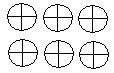Name: ___________________Date:___________________

 Email us to get an instant 20% discount on highly effective K-12 Math & English kwizNET Programs!

### Grade 2 - Mathematics7.9 Multiplication Review Test

 Q 1: Write the multiplication form for 5 + 5 + 5 + 5 + 5 + 55 X 5 = 255 X 6 = 305 + 5 = 10 Q 2: Write the multiplication form for 3 + 3 + 3 + 33 X 4 = 123 X 5 = 20120 r23 + 4 = 7 Q 3: Use the figure to find 3 times 2.674 Q 4: What are the factors of the multiplication 76 x 4 = 30476 and 44 and 30476 and 304 Q 5: What is the product of the multiplication 83 x 1 =83303880 Q 6: 3 x 7 =4none of the above2010 Q 7: What are the factors of the multiplication 6 x 3 = 1818 and 36 and 36 and 18 Q 8: 3 x 9 =24282527 Question 9: This question is available to subscribers only! Question 10: This question is available to subscribers only!